# Difference between revisions of "Chain rule for differentiation"

Jump to: navigation, search

This article is about a differentiation rule, i.e., a rule for differentiating a function expressed in terms of other functions whose derivatives are known.
View other differentiation rules

## Statement for two functions

The chain rule is stated in many versions:

Version type Statement
specific point, named functions Suppose$f$ and$g$ are functions such that$g$ is differentiable at a point$x = x_0$, and$f$ is differentiable at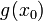$g(x_0)$. Then the composite$f \circ g$ is differentiable at$x_0$, and we have:$\! \frac{d}{dx}[f(g(x))]|_{x = x_0} = f'(g(x_0))g'(x_0)$
generic point, named functions, point notation Suppose$f$ and$g$ are functions of one variable. Then, we have$\! \frac{d}{dx}[f(g(x))] = f'(g(x))g'(x)$ wherever the right side expression makes sense.
generic point, named functions, point-free notation Suppose$f$ and$g$ are functions of one variable. Then,$\! (f \circ g)' = (f' \circ g) \cdot g'$ where the right side expression makes sense, where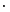$\cdot$ denotes the pointwise product of functions.
pure Leibniz notation Suppose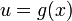$u = g(x)$ is a function of$x$ and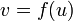$v = f(u)$ is a function of$u$. Then,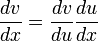$\frac{dv}{dx} = \frac{dv}{du}\frac{du}{dx}$
MORE ON THE WAY THIS DEFINITION OR FACT IS PRESENTED: We first present the version that deals with a specific point (typically with a$\{ \}_0$ subscript) in the domain of the relevant functions, and then discuss the version that deals with a point that is free to move in the domain, by dropping the subscript. Why do we do this?
The purpose of the specific point version is to emphasize that the point is fixed for the duration of the definition, i.e., it does not move around while we are defining the construct or applying the fact. However, the definition or fact applies not just for a single point but for all points satisfying certain criteria, and thus we can get further interesting perspectives on it by varying the point we are considering. This is the purpose of the second, generic point version.

### One-sided version

A one-sided version of sorts holds, but we need to be careful, since we want the direction of differentiability of$f$ to be the same as the direction of approach of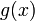$g(x)$ to$g(x_0)$. The following are true:

• We require$g$, the function composed first, to be left differentiable at$x_0$ and require$f$ to be differentiable at$g(x_0)$. Then, the left hand derivative of$f \circ g$ at$x_0$ is$f'(g(x_0))$ times the left hand derivative of$g$ at$x_0$.
• We require$g$, the function composed first, to be right differentiable at$x_0$ and require$f$ to be differentiable at$g(x_0)$. Then, the right hand derivative of$f \circ g$ at$x_0$ is$f'(g(x_0))$ times the right hand derivative of$g$ at$x_0$.
• We require$g$ to be left differentiable at$x_0$,$f$ to be left differentiable at$g(x_0)$, and we require$g$ to be an increasing function. Then,$f \circ g$ is left differentiable at$x_0$ and the left hand derivative is the left hand derivative of$f$ at$g(x_0)$ times the left hand derivative of$g$ at$x_0$.
• We require$g$ to be right differentiable at$x_0$,$f$ to be right differentiable at$g(x_0)$, and we require$g$ to be an increasing function. Then,$f \circ g$ is right differentiable at$x_0$ and the right hand derivative is the right hand derivative of$f$ at$g(x_0)$ times the left hand derivative of$g$ at$x_0$.
• We require$g$ to be left differentiable at$x_0$,$f$ to be right differentiable at$g(x_0)$, and we require$g$ to be a decreasing function. Then,$f \circ g$ is left differentiable at$x_0$ and the left hand derivative is the right hand derivative of$f$ at$g(x_0)$ times the left hand derivative of$g$ at$x_0$.
• We require$g$ to be right differentiable at$x_0$,$f$ to be left differentiable at$g(x_0)$, and we require$g$ to be a decreasing function. Then,$f \circ g$ is right differentiable at$x_0$ and the right hand derivative is the left hand derivative of$f$ at$g(x_0)$ times the left hand derivative of$g$ at$x_0$.

## Statement for multiple functions

Suppose$f_1,f_2,\dots,f_n$ are functions. Then, the following is true wherever the right side makes sense: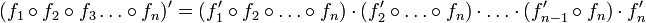$(f_1 \circ f_2 \circ f_3 \dots \circ f_n)' = (f_1' \circ f_2 \circ \dots \circ f_n) \cdot (f_2' \circ \dots \circ f_n) \cdot \dots \cdot (f_{n-1}' \circ f_n) \cdot f_n'$

For instance, in the case$n = 3$, we get:$(f_1 \circ f_2 \circ f_3)' = (f_1' \circ f_2 \circ f_3) \cdot (f_2' \circ f_3) \cdot f_3'$

In point notation, this is:$\! \frac{d}{dx}[f_1(f_2(f_3(x)))] = f_1'(f_2(f_3(x))f_2'(f_3(x))f_3'(x)$